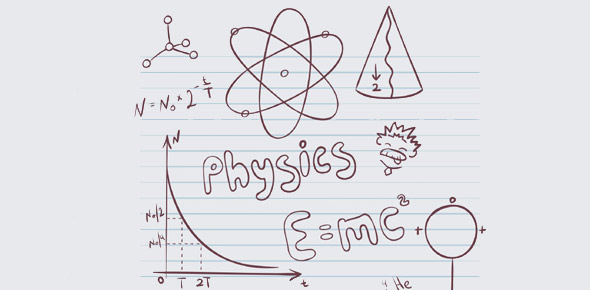# Physics Chapter 24

28 Questions | Total Attempts: 322SettingsPhysics Chapter 24

Related Topics
• 1.
The source of all magnetism is
• A.

Tiny pieces of iron

• B.

Tiny domains of aligned atoms

• C.

Ferromagnetic materials

• D.

Moving electric charge

• E.

None of these

• 2.
Magnetic compasses apparently were first used by
• A.

Columbus

• B.

Greeks

• C.

Australian aborigines

• D.

Vikings

• E.

Pigeons, then Chinese

• 3.
Moving electric charges will interact with
• A.

An electric field

• B.

A magnetic field

• C.

Both of these

• D.

None of these

• 4.
If a steady magnetic field exerts a force on a moving charge, that force is directed
• A.

Opposite the direction of the motion

• B.

In the direction of the motion

• C.

At right angles to the direction of the motion

• 5.
If a steady magnetic field exerts a force on a moving charge, that force is directed
• A.

In the direction of the field

• B.

Opposite the direction of the field

• C.

At right angles to the direction of the field

• 6.
An iron rod becomes magnetic when
• A.

Positive ions accumulate at one end and negative ions at the other end

• B.

It atoms are aligned having plus charges on one side and negative charges on the other

• C.

The net spins of its electrons are in the same direction

• D.

Its electrons stop moving and point in the same direction

• E.

None of these

• 7.
Like kinds of magnetic poles repel while unlike kinds of magnetic poles
• A.

Attract

• B.

Repel also

• C.

May attract or repel

• 8.
Several paper clips dangle from the north pole of a magnet. the induced pole in the bottom of the lowermost paper clip is a
• A.

North pole

• B.

South pole

• C.

North or south pole-no difference really

• 9.
An iron nail is more strongly attracted to the
• A.

North pole of a magnet

• B.

South pole of a magnet

• C.

North or south pole- no difference really

• 10.
Surrounding every moving electron is
• A.

A magnetic field

• B.

An electric field

• C.

Both of these

• D.

None of these

• 11.
Magnetism is due to the motion of electrons as they
• A.

Move around the nucleus

• B.

Spin on their axes

• C.

Both of these

• D.

None of these

• 12.
Magnetic domains normally occur in
• A.

Iron

• B.

Copper

• C.

Silver

• D.

All of these

• E.

None of these

• 13.
Magnetic field lines about a current-carrying wire
• A.

• B.

Circle the wire in closed loops

• C.

Both of these

• D.

Neither of these

• 14.
• A.

The same as the magnetic field direction

• B.

Exactly opposite tot he magnetic field direction

• C.

Perpendicular to the magnetic field direction

• D.

At an angle other than 90 degrees tot eh magnetic field direction

• E.

None of these

• 15.
The intensity of cosmic rays bombarding the Earth's surface is largest at the
• A.

Poles

• B.

Mid-latitudes

• C.

Equator

• 16.
If we think of the Earth as a magnet, its north (SEEKING) pole is nearest
• A.

The Hudson Bay region of Canada

• B.

Australia

• C.

Both of these

• 17.
Which pole of a compass needle points to a south pole of a magnet?
• A.

North pole

• B.

South pole

• C.

Both of these

• 18.
Pigeons navigate primarily by
• A.

A good memory

• B.

A keen sense of smell

• C.

• D.

Ultra-high pitched sounds

• E.

None of these

• 19.
Magnet A has twice the magnetic field strength of Magnet B and at a certain distance pulls on magnet B with a force of 100 N. the amount of force that magnet A exerts on magnet B is
• A.

• B.

Exactly 100 N

• C.

• 20.
Compared to the huge force that attracts an iron tack to a strong magnet, the force that the tack exerts on the magnet is
• A.

Relatively small

• B.

Equally huge

• C.

• 21.
An electron is hot from the right, toward a spot just between the ends of the magnet. the electron
• A.

Is unaffected by the field

• B.

Is attracted to one of the poles,and repelled from the other

• C.

Is repelled by both poles, and therefore is turned back

• D.

Is deflected into the plane of the page

• E.

Accelerates straight toward the spot

• 22.
If a compass is moved form northern hemisphere to the southern hemisphere, its magnetic needle will change direction
• A.

By 180 degrees

• B.

Depending on where the measurement is taken

• C.

By 90 degrees

• D.

Hardly at all

• E.

None of these

• 23.
A possible cause for the existence of the Earth's magnetic field is
• A.

Moving charges in the liquid part of the Earth's core

• B.

Great numbers of very slow moving charges in the Earth

• C.

Convection currents in the liquid part of the Earth's core

• D.

All of these

• E.

None of these

• 24.
Outside a magnet, magnetic field lines are conventionally drawn from
• A.

North to south

• B.

South to north

• C.

Either way

• 25.
Which force field can increase a moving electron's speed?
• A.

Electric field

• B.

Magnetic filed

• C.

Bother of these

• D.

None of these